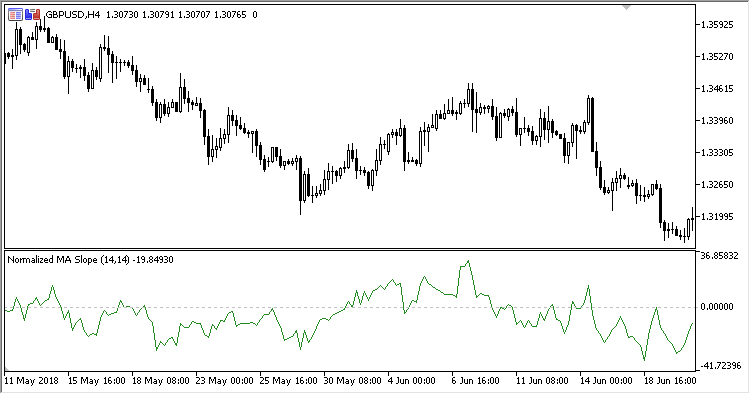Interesting script?
So post a link to it -
let others appraise it

You liked the script? Try it in the MetaTrader 5 terminal# Normalized_Moving_Average_Slope - indicator for MetaTrader 5

Views:
1251
Rating:
Published:
2018.12.18 19:15

Normalized Moving Average Slope oscillator calculates the slope of the moving average in % of ATR.

It has four inputs:

• MA period - MA calculation period
• ATR period - ATR calculation period
• Method - MA calculation method
• Applied price - MA calculation price

Calculation:

NMAS = 100.0 * (MA-PrevMA) / ATR

where:

MA - MA(Applied price, MA period, Method)
ATR - ATR(ATR period)Translated from Russian by MetaQuotes Software Corp.
Original code: https://www.mql5.com/ru/code/22508SineWMA

Sine Weighted MA indicatorSmoothed_RSI

Smoothed RSI indicatorMACD_Flat_Trend

MACD Flat Trend indicatorFoM

Freedom of Movement indicator